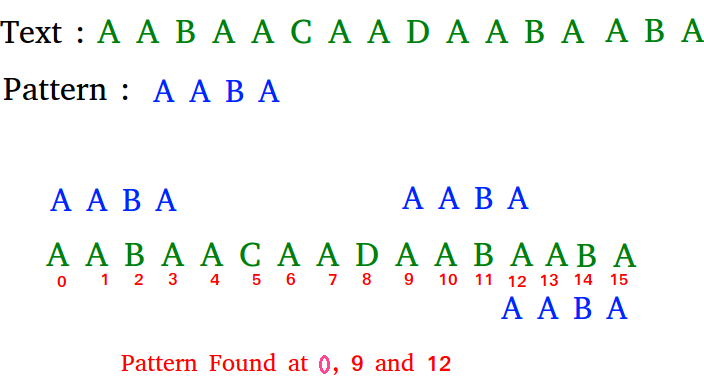Related Articles

# C# Program for Naive algorithm for Pattern Searching

• Last Updated : 11 Dec, 2018

Given a text txt[0..n-1] and a pattern pat[0..m-1], write a function search(char pat[], char txt[]) that prints all occurrences of pat[] in txt[]. You may assume that n > m.

Examples:

```Input:  txt[] = "THIS IS A TEST TEXT"
pat[] = "TEST"
Output: Pattern found at index 10

pat[] =  "AABA"
Output: Pattern found at index 0
Pattern found at index 9
Pattern found at index 12```

Pattern searching is an important problem in computer science. When we do search for a string in notepad/word file or browser or database, pattern searching algorithms are used to show the search results.

## C#

 `// C# program for Naive Pattern Searching``using` `System;`` ` `class` `GFG {`` ` `    ``public` `static` `void` `search(String txt, String pat)``    ``{``        ``int` `M = pat.Length;``        ``int` `N = txt.Length;`` ` `        ``/* A loop to slide pat one by one */``        ``for` `(``int` `i = 0; i <= N - M; i++) {``            ``int` `j;`` ` `            ``/* For current index i, check for pattern ``            ``match */``            ``for` `(j = 0; j < M; j++)``                ``if` `(txt[i + j] != pat[j])``                    ``break``;`` ` `            ``// if pat[0...M-1] = txt[i, i+1, ...i+M-1]``            ``if` `(j == M)``                ``Console.WriteLine(``"Pattern found at index "` `+ i);``        ``}``    ``}`` ` `    ``// Driver code``    ``public` `static` `void` `Main()``    ``{``        ``String txt = ``"AABAACAADAABAAABAA"``;``        ``String pat = ``"AABA"``;``        ``search(txt, pat);``    ``}``}``// This code is Contributed by Sam007`
Output:
```Pattern found at index 0
Pattern found at index 9
Pattern found at index 13
```

Please refer complete article on Naive algorithm for Pattern Searching for more details!

My Personal Notes arrow_drop_up